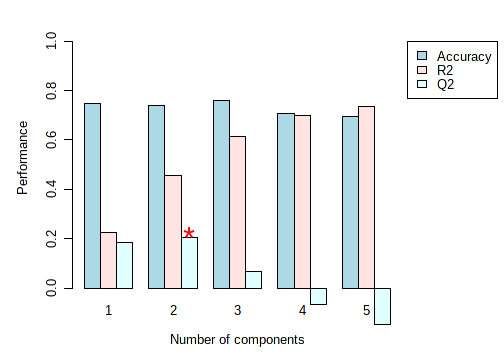# What is Q2 value (PLS-DA) and why my Q2 value is negative?

Q2 is an estimate of the predictive ability of the model, and is calculated via cross-validation (CV). In each CV, the predicted data are compared with the original data, and the sum of squared errors is calculated. The prediction error is then summed over all samples (Predicted Residual Sum of Squares or PRESS). For convenience, the PRESS is divided by the initial sum of squares and subtracted from 1 to resemble the scale of the R2.

Good predictions will have low PRESS or high Q2. It is possible to have negative Q2 (due to the subtraction step) , which means that your model is not at all predictive or is overfitted. For more details, refer to an excellent paper by (Szymańska, et al).

An example plot from PLS-DA CV result is shown below (note, Q2 becomes negative when top 4 or 5 components are used, indicating overfitting occurs)1 Like

Hello Dr jeff.xia!

Now that you have said:

Q2 becomes negative when top 4 or 5 components are used

How can you select how many components sould be used for building the PLS-DA model in MetaboAnalyst?

I have used other chemometrics softwares that allow selecting the number of components for building the model, but I have not found this option in MetaboAnalyst.